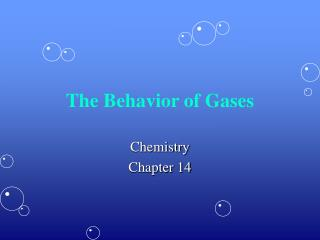DownloadDownload PresentationThe Behavior of Gases

# The Behavior of Gases

Download Presentation## The Behavior of Gases

- - - - - - - - - - - - - - - - - - - - - - - - - - - E N D - - - - - - - - - - - - - - - - - - - - - - - - - - -
##### Presentation Transcript

1. The Behavior of Gases Chemistry Chapter 14

2. Properties of Gases • Gases are easily compressed, or squeezed, into a smaller space. • Compressibilityis a measure of how much the volume of matter decreases under pressure. • Gases are easily compressed because of the empty space between the particles in a gas. • The amount of gas- moles (n),volume – liter (V), and temperature – kelvin (T)are factors that affectgas pressure – kilopascal (P).

3. Variables & Pressure

4. The Gas Laws • The laws that describe how gases behave depending upon the four variables: * Temp (T) * Volume (V) * Pressure (P) * Amount of gas (n) • The four gas laws we will be discussing: • Boyle’s Law • Charles’ Law • Gay-Lussac’s Law • The Combined Gas Laws

5. Boyle’s Law • How would an increase in pressure affect the volume of a contained gas? • If the temperature is constant, as the pressure of a gas increases, the volume decreases. In turn as the pressure decreases the volume increases. • Boyle’s Law states that for a given mass of gas at constant temp, the volume of gas varies inversely with the pressure. P1 × V1 = P2 × V2

6. Charles’ Law • As the temperature of an enclosed gas increases, the volume increases, if the pressure is constant. • Charles studied effects of temp on the volume of gas at constant pressure. • Observations are summarized in Charles’ Law, which states, that the volume of a fixed mass of gas is directly proportional to its Kelvin temperature if the pressure is kept constant.

7. Gay-Lussac’s Law • As the temperature of an enclosed gas increases, the pressure increases, if the volume is constant. • Gay-Lussac’s Law states that the pressure of a gas is directly proportional to the Kelvin temp if the volume remains constant. Because Gay-Lussac’s law involves direct proportions, the ratios P1/T1 and P2/T2 are equal. Thus Gay-Lussac’s law can be written:

8. The Combined Gas Laws • Combines Boyle’s, Charles’, and Gay-Lussac’s Laws into a single expression. • This allows you to do calculations for situations in which only the amount of gas (moles) is constant.

9. Ideal Gases • To calculate the number of moles of a contained gas requires an expression that contains the variablen. • The combined gas law can be modified to include # of moles. • This equation shows that (P x V) / (T x n) is a constant. • This constant holds for ideal gases (gases that conform to the gas laws).

10. The Ideal Gas Constant (R) • If you know the values for P, V, T, and n for one set of conditions, you can calculate a value for the constant. • Recall that 1 mol of every gas occupies 22.4 L at STP (101.3 kPa and 273 K). You can use these values to find the value of the constant, which has the symbol R and is called the ideal gas constant. • Insert the values of P, V, T, and n into (P × V)/(T × n). • The ideal gas constant (R) has the value 8.31 (L·kPa)/(K·mol).

11. The Ideal Gas Law • The gas law that includes all four (4) variables (P, V, T, and n) is called the Ideal Gas Law. P x V = n x R x T Or PV = nRT

12. Real vs. IdealGases • Ideal gases don’t exist! • However, at many conditions of temp & pressure real gases behave very much like an ideal gas. • Real gases differ most from an ideal gas at low temps and high pressures.

13. Mixtures of Gas • In a mixture of gases, the contribution each gas makes to the total pressure is calledpartial pressure. • Dalton’s Law – at constant volume & temp, the total pressure exerted by a mixture of gases is equal to the sum of the partial pressures of each component gas. Ptotal = P1 + P2 + P3 +….

14. How Gases Move • Diffusion is the tendency of molecules to move toward areas of lower concentration, until concentration is uniform. • Effusion involves gas escaping through a tiny hole in its container. • Gases of lower molar mass diffuse & Effuse faster that gases of higher molar mass. • Graham’s Law of Effusion – the rate of effusion of a gas is inversely proportional to the square root of the gases molar mass. • Another words the gas with the lower atomic mass effuses fastest.

15. Graham’s Law (continued) • Graham’s Law can be written as follows for two gases, A and B: • This expression compares the rates of effusion of any two gases, at the same temp & pressure.